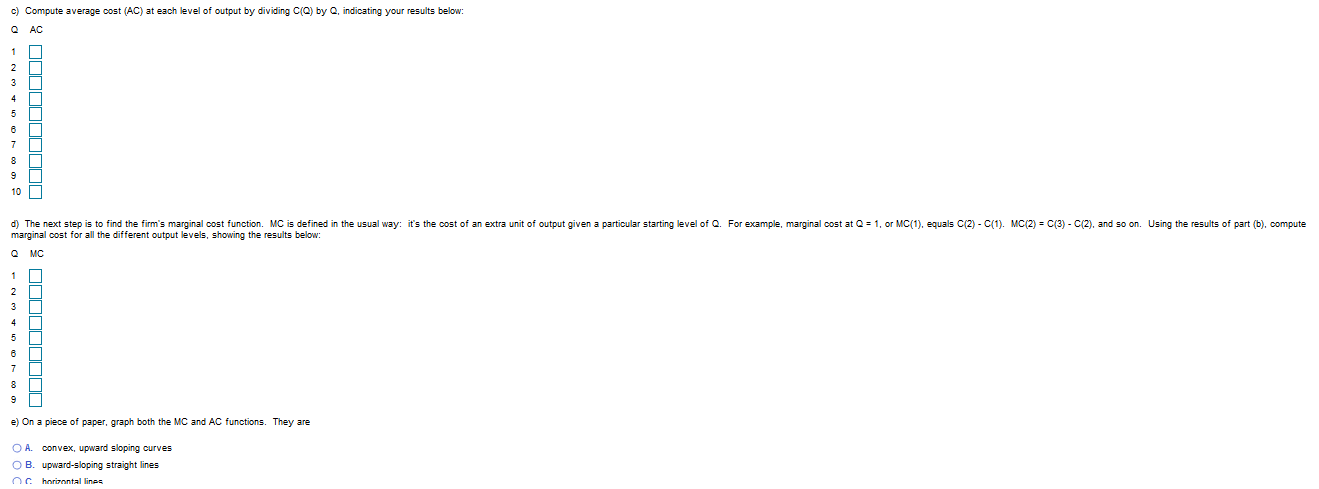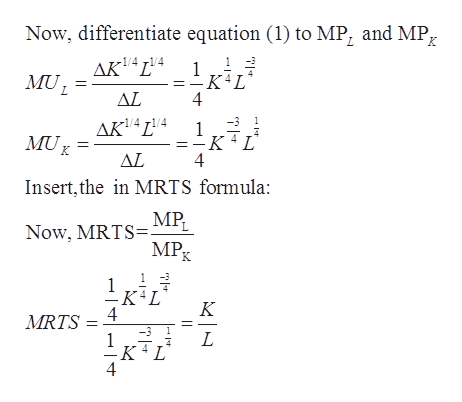# c) Compute average cost (AC) at each level of output by dividing C(Q) by Q, indicating your results below:ACd) The next step is to find the firm's marginal cost function. MC is defined in the usual way: it's the cost of an extra unit of output given a particular starting level of Q. For example, marginal cost at Q 1, or MC(1), equals C(2)marginal cost for all the different output levels, showing the results below:C(1). MC(2) C(3) - C(2), and so on. Using the results of part (b). computeQMC12e) On a piece of paper, graph both the MC and AC functions. They areO A.convex, upward sloping curvesO B.upward-sloping straight linesO horizontal lines

Question
61 views
Suppose a firm uses capital​ (K) and labor​ (L) to produce widgets according to the production function Q​ =
K​^(1/4)L​^(1/4)
​ =
​(KL)^(​1/4)​,
where Q is output. The goal in this problem is to find the​ firm's total and marginal cost functions. In order to derive the cost​ function, the first step is to find the location of the​ cost-minimizing bundle on any given isoquant. To get a numerical​ answer, recall that the slope of an isoquant is
​-MPL​/MPK
​ (K is on the vertical​ axis). Using​ calculus, it can be shown that this slope formula reduces to​ -K/L. For​ example, consider the input bundle with K​ = 8, L​ = 2 on the isoquant with Q​ = 2​ (note that 2​ =
16​1/4
​ ). The formula indicates that the slope at this point is​ -4 =​ -8/2. In​ fact, the formula tells us that regardless of which isoquant an input bundle is​ on, the slope will be​ -4 as long as the ratio of K to L is​ 4:1. ​ Similarly, the isoquant slope will be​ -1 whenever the ratio of K to L is​ 1:1.

In answering the following​ questions, use integer values only​ (don't enter unneeded decimal​ points).help_outlineImage Transcriptionclosec) Compute average cost (AC) at each level of output by dividing C(Q) by Q, indicating your results below: AC d) The next step is to find the firm's marginal cost function. MC is defined in the usual way: it's the cost of an extra unit of output given a particular starting level of Q. For example, marginal cost at Q 1, or MC(1), equals C(2) marginal cost for all the different output levels, showing the results below: C(1). MC(2) C(3) - C(2), and so on. Using the results of part (b). compute QMC 1 2 e) On a piece of paper, graph both the MC and AC functions. They are O A. convex, upward sloping curves O B. upward-sloping straight lines O horizontal lines fullscreen
check_circle

Step 1

Step1. There was some technical problem while uploading the question. that is why I am writing the sequence of the solution and the solution is after this step. Moreover, the E part is incomplete. So I am avoiding that part.

Step 2

Step2.

Given, the production function:

Step 3

step 3. Differentiate ...help_outlineImage TranscriptioncloseNow, differentiate equation (1) to MP, and MP, K AKL4 MUL 1 KAL 4 ΔL -1/4 r1/4 1 KL 4 MU AL Insert, the in MRTS formula: MPL Now, MRTS= MPg K 4 MRTS 1 K L 4 fullscreen

### Want to see the full answer?

See Solution

#### Want to see this answer and more?

Solutions are written by subject experts who are available 24/7. Questions are typically answered within 1 hour.*

See Solution
*Response times may vary by subject and question.
Tagged in